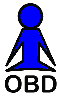MAP- and MAF-Based Air/Fuel Flow Calculator

 Air/Fuel Ratio Limits 6.0:1    9.0:1  11.5:1  12.5:1  13.2:1  14.7:1  15.5:1  16.2:1  18-22:1 Rich run limit   Low power, black smoke   Rich best torque at WOT   Safe best power at WOT   Lean best torque at WOT   Chemically ideal   Lean light load, part throttle   Best economy, part throttle   Lean run limit

Making use of the Ideal Gas Law, a synthetic variable called IMAP can be used to estimate the mass air-flow (MAF) of an internal combustion vehicle, much like a MAF sensor. However, in order to make this work, the engine displacement and volumetric efficiency of the engine must be provided.

IMAP is integrated by the firmware using the following formula:

```        IMAP = RPM * MAP / IAT / 2
```
where RPM is engine speed in revolutions per minute, MAP (manifold absolute pressure) is measured in KPa, and IAT (intake air temperature) is measured in degrees Kelvin. This integrated value can be converted into total air flow (grams) using the following formula:
```        (grams of air) = (IMAP/60)*(Vol Eff/100)*(Eng Disp)*(MM Air)/(R)
```
where R is 8.314 J/°K/mole and the average molecular mass of air (MM) is 28.97 g/mole. Note that in the above formula the volumetric efficiency of the engine is measured in percent and the displacement is in liters.

Once we have MAF integrated over time, we can calculate fuel flow using a specific air/fuel mixing ratio (A/F). A/F can range from 6 to 22. The total mass of air which flows through the engine (as measured by the MAF sensor or calculated using MAP, RPM and IAT) is divided by the A/F ratio (e.g., 14.7) to derive the total mass of fuel that has been burned.

If the air mass is measured in grams, then the calculated amount of fuel will also be in grams. Using an average density of gasoline of 6.17 pounds per gallon and knowing that there are 454 grams per pound, then dividing the total fuel mass in grams by (454 * 6.17) yields the total number of gallons of fuel burned for a given amount of air (as measured by the MAF sensor).

Fuel consumed can be estimated from integrated MAF using the following formula:

```        (gallons of fuel) = (grams of air) / (air/fuel ratio) / 6.17 / 454
```
Knowing the total fuel consumed and the distance traveled, the fuel consumption rate in MPG (miles per gallon) then can be calculated.
 MAF-Based Fuel MPG Calculator Total Mass Air Flow (g) Odometer (mi) A/F Ratio Fuel Used Mileage Start Finish Start Finish Gallons MPG

 MAP-Based Fuel MPG Calculator IMAP (MAP*RPM/IAT/2) Odometer (mi) Eng Disp Vol Eff A/F Ratio Fuel Used Mileage Start Finish Start Finish Liters % Gallons MPG